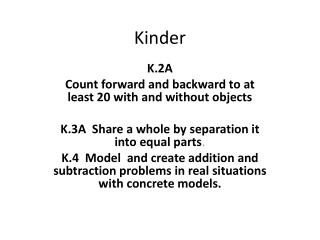Download PresentationKinder# Kinder - PowerPoint PPT Presentation

Download Presentation##### Kinder

Download Policy: Content on the Website is provided to you AS IS for your information and personal use and may not be sold / licensed / shared on other websites without getting consent from its author. While downloading, if for some reason you are not able to download a presentation, the publisher may have deleted the file from their server.

- - - - - - - - - - - - - - - - - - - - - - - - - - - E N D - - - - - - - - - - - - - - - - - - - - - - - - - - -
##### Presentation Transcript

1. Kinder K.2A Count forward and backward to at least 20 with and without objects K.3A Share a whole by separation it into equal parts. K.4 Model and create addition and subtraction problems in real situations with concrete models.

2. 1st Grade • 1.2A Recognize instantly the quantity of structured arrangements such as seen on a die or a ten frame. • 1.2B Use appropriate language to describe part of a set such as three out of the eight crayons are red. • 1.7A Estimate and measure length using non-standard units such as paper clips or sides of color tiles.

3. 2nd Grade • 2.2A Use concrete and pictorial models to compose and decompose numbers up to 1,200 as a sum of so many thousands, hundreds, tens, and ones, in more than one way. • 2.3D Determine the value of a collection of coins. • Describe attributes (the number of vertices, faces, edges, sides) of two dimensional geometric figures such as circles, polygons, spheres, cones, cylinders, prisms, and pyramids.

4. 3rd Grade • 3.2A Compose and decompose numbers up to 100,000 as a sum of so many ten thousands, so many thousands, so many hundreds, so many tens, so many ones, in more than one way, using objects, pictorial models, and numbers including expanded notation, as appropriate. • 3.8A Identify, classify, and describe two-dimensional and three-dimensional geometric figures by their attributes. The student compares two dimensional figures, three-dimensional figures, or both by their attributes using formal geometric vocabulary. • 3.11B Use standard units to find perimeter of a shape.

5. 4th Grade • 4.2A Interpret the value of each place-value position as ten times the position to the right and as one-tenth of the value of the place to its left. • 4.13A Interpret bar graphs • 4.7A Describe the relationship between two sets of data such as ordered pairs in a table.

6. 5th Grade • 5.2A Interpret the value of each place-value position as one-tenth of the value of the place to its left or as ten times the value of the place to the right. • 5.10.C select and use appropriate units and formulas to measure length, perimeter, area, and volume • 5.5.A describe the relationship between sets of data in graphic organizers such as lists, tables, charts, and diagrams

7. 6th Grade • 6.2A Classify whole numbers, integers, and rational numbers using a visual representation such as a Venn Diagram to describe the relationships between sets of numbers.